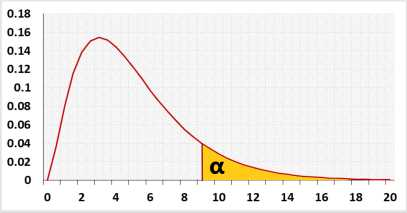# D'Agostino-Pearson Test Calculator

D'Agostino-Pearson Test, Skewness test, Kurtosis test.

When entering data, press comma , , Space or Enter after each value.
You may copy and paste data from Excel or Google Sheets. Leaving empty cells is okay. The tool doesn't count empty cells or non-numeric cells.

## Information

The following statistical tests compare the shape of the distribution to the symmetric normal distribution.
The skewness test checks the symmetric level, the kurtosis test checks the distribution of the data over the tails and the middle, While D'Agostino-Pearson combines the skewness test and the kurtosis test, hence used as a normality test.

### D'Agostino-Pearson test

The D'Agostino-Pearson test checks if the shape of the distribution is similar to the shape of the normal distribution.
This is a combination of the skewness test and the kurtosis test.
The D'Agostino-Pearson is not the most powerful test, but you may use it for any distribution.
For a normality test, if the data doesn't have repeated values we recommend using the Shapiro-Wilk test.
If the data contains repeated values we recommend using the D'Agostino-Pearson test.

#### Target

Tests if the distribution model fits the observations
The tool combines the following methods:
1. A formal normality test: D'Agostino-Pearson test.
2. Graphical methods: QQ-Plot chart and Histogram.

### Skewness test

The skewness test tests the null assumption that the data distribution is symmetric.

### Kurtosis test

The kurtosis test tests the null assumption that the kurtosis equals zero (kurtosis of the normal distribution).

The following table will be changed when you change the test or tail.

Hypotheses
H0: Normal distribution
H1: Other distribution
Test statistic
χ2(2) = Z2kurtosis + Z2skewness
Distribution## Normality effect size

We use the D statistic as effect size
We know only the sample effect size!.
We define the following effect levels of the effect size:

• Small - the effect size of Chi-squared(df=20)
• Medium - the effect size of Chi-squared(df=6)
• Large - the effect size of Chi-squared(df=3)
For example, Small is any value between D of Chi-squared(df=20) abd D of Chi-squared(df=6).
For the same distribution D depends on the sample size, we calculated the D per the following sample sizes: 10, 20, 40, 80, 160, 320, 640, 1280
We calculated the effect size of Chi-squared sample data using a simulation with 50,000 repeats.. The effect level is only wild rule of thumb, we still recommend to look at the Q-Q plot.

## Reference

D'agostino, R. B., Belanger, A., & D'Agostino Jr, R. B. (1990). A suggestion for using powerful and informative tests of normality. The American Statistician, 44(4), 316-321.